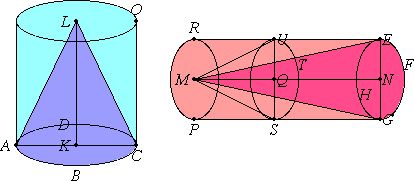# Proposition 15

In equal cones and cylinders the bases are reciprocally proportional to the heights; and those cones and cylinders in which the bases are reciprocally proportional to the heights are equal.

Let there be equal cones and cylinders with the circular bases ABCD and EFGH. Let AC and EG be the diameters of the bases, and KL and MN the axes, which are also the heights of the cones or cylinders

Complete the cylinders AO and EP.

I say that in the cylinders AO and EP the bases are reciprocally proportional to the heights, that is, the base ABCD is to the base EFGH as the height MN is to the height KL.For the height LK is either equal to the height MN or unequal.

First, let it be equal.

XII.11

Now the cylinder AO also equals the cylinder EP. But cones and cylinders of the same height are to one another as their bases, therefore the base ABCD equals the base EFGH.

Hence, reciprocally, the base ABCD is to the base EFGH as the height MN is to the height KL.

Next, let the height LK be unequal to MN, and let MN be greater.

Cut QN off the height MN equal to KL. Through the point Q let the cylinder EP be cut by the plane TUS parallel to the planes of the circles EFGH and RP. Erect the cylinder ES from the circle EFGH as base and with height NQ.

V.7

Now, since the cylinder AO equals the cylinder EP, therefore the cylinder AO is to the cylinder ES as the cylinder EP is to the cylinder ES.

But the cylinder AO is to the cylinder ES as the base ABCD is to the base EFGH, for the cylinders AO and ES are of the same height. And the cylinder EP is to the cylinder ES as the height MN is to the height QN, for the cylinder EP is cut by a plane parallel to its opposite planes. Therefore the base ABCD is to the base EFGH as the height MN is to the height QN.

But the height QN equals the height KL, therefore the base ABCD is to the base EFGH as the height MN is to the height KL.

Therefore in the cylinders AO and EP the bases are reciprocally proportional to the heights.

Next, in the cylinders AO and EP let the bases be reciprocally proportional to the heights, that is, as the base ABCD is to the base EFGH, so let the height MN be to the height KL.

I say that the cylinder AO equals the cylinder EP.

With the same construction, since the base ABCD is to the base EFGH as the height MN is to the height KL, and the height KL equals the height QN, therefore the base ABCD is to the base EFGH as the height MN is to the height QN.

But the base ABCD is to the base EFGH as the cylinder AO is to the cylinder ES, for they have the same height. And the height MN is to QN as the cylinder EP is to the cylinder ES, therefore the cylinder AO is to the cylinder ES as the cylinder EP is to the cylinder ES.

V.9

Therefore the cylinder AO equals the cylinder EP.

XII.10

And the same is true for the cones also.

Therefore, in equal cones and cylinders the bases are reciprocally proportional to the heights; and those cones and cylinders in which the bases are reciprocally proportional to the heights are equal.

Q.E.D.

## Guide

This proof of this proposition applies to a more general situation than cones and cylinders. Whenever a magnitude x is proportional to two other magnitudes y and z, that is to say when y is fixed then x is proportional to z and when x is fixed then y is proportional to z, it follows that when x is fixed then y and z are reciprocally proportional.

This proposition completes the theory of the volumes of cones and cylinders. The remaining three propositions in this book concern the volume of spheres.